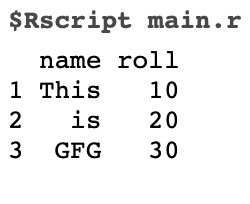How to export a DataFrame to Excel File in R ?

• Last Updated : 21 Apr, 2021

It is a frequent requirement to save our dataframe for portability after working on it on the auxiliary memory of the computer system using R Programming Language. In this article, we will be using writexl package to export our dataframe to excel(.xlsx). The write_xlsx() function of the writexl package is used to export our dataframe to an Excel file.

Getting Started

The writexl is a simple package that contains a function write_xlsx() function which is used to write a dataframe to an Excel (.xlsx) file.

Installation

Packages are installed in R by using the install.packages() function. To install a package pass the name of the package/library to the install.packages() function.

We can install the writexl package by running the command below in the R console.

install.packages("writexl")

Export the dataframe to excel

Example 1: In the first line of the code below, we have used library(“writexl”) function to load the package named -> “writexl”. We have then used write_xlsx() function of the writexl library to export the dataframe to an Excel file. In the below example, our write_xlsx() function takes 2 arguments, the first argument is the dataframe itself which is to be converted to an Excel file, the second argument is the path with “file_name.xlsx” which specifies the location where our Excel file will be saved with specified file_name.

To create a “.xlsx” with (multiple) named sheets, simply set “df” to a named list of data frames.

Syntax: write_xlsx(dataframe_name, “path\\file_name.xlsx”)

Code:

R

 # loads the packagelibrary("writexl")   # sample dataframedf <- data.frame(name = c("This", "is", "GFG"),                 roll = c(10,20,30))df  # saves the dataframe at the specified# pathwrite_xlsx(df,"MY_PATH\df.xlsx")

Output:Our dataframe gets exported in the form of an Excel file to the specified location or path.

Example 2:

The only difference in this example from the previous examples is the arguments, in the example code below we have passed 2 extra arguments which help us have more control over the formatting of the Excel file. Those arguments and their usage are:

• col_names: write column names at the top of the Excel file(.xlsx)
• format_headers: make the column names(col_names) in the Excel file(.xlsx) centered and bold

Syntax:

write_xlsx(
df,
"path\\file_name.xlsx"),
col_names = TRUE,
)

Code:

R

 # loads the packagelibrary("writexl")   # sample dataframedf <- data.frame(name = c("This", "is", "GFG"),                 roll = c(10,20,30))df  # saves the dataframe at the specified pathwrite_xlsx(        df,"My_path\\df.xlsx",        col_names = TRUE,        format_headers = TRUE)

Output:Our dataframe gets exported in the form of an excel file to the specified location or path.

Export the dataframe to a CSV

The write.csv() is an inbuilt function in R, we do not require installing any additional library for using this function.

In the code below, our write.csv() function takes 2 arguments, the first argument is the dataframe itself which is to be converted to a CSV file, the second argument is the path with “file_name.csv” which specifies the location where our CSV file will be saved with specified file_name.

Syntax: write.csv(dataFrame_name, “path\\file_name.csv”)

Code:

R

 # sample dataframedf <- data.frame(name = c("This", "is", "GFG"),                 roll = c(10,20,30))df  # saves the dataframe at the specified pathwrite.csv(df,"My_Path\\df.csv")

Output:My Personal Notes arrow_drop_up